# Artificial Neural Network - Building Blocks

Processing of ANN depends upon the following three building blocks −

• Network Topology
• Adjustments of Weights or Learning
• Activation Functions

In this chapter, we will discuss in detail about these three building blocks of ANN

## Network Topology

A network topology is the arrangement of a network along with its nodes and connecting lines. According to the topology, ANN can be classified as the following kinds −

### Feedforward Network

It is a non-recurrent network having processing units/nodes in layers and all the nodes in a layer are connected with the nodes of the previous layers. The connection has different weights upon them. There is no feedback loop means the signal can only flow in one direction, from input to output. It may be divided into the following two types −

• Single layer feedforward network − The concept is of feedforward ANN having only one weighted layer. In other words, we can say the input layer is fully connected to the output layer.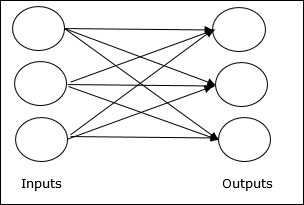• Multilayer feedforward network − The concept is of feedforward ANN having more than one weighted layer. As this network has one or more layers between the input and the output layer, it is called hidden layers.### Feedback Network

As the name suggests, a feedback network has feedback paths, which means the signal can flow in both directions using loops. This makes it a non-linear dynamic system, which changes continuously until it reaches a state of equilibrium. It may be divided into the following types −

• Recurrent networks − They are feedback networks with closed loops. Following are the two types of recurrent networks.

• Fully recurrent network − It is the simplest neural network architecture because all nodes are connected to all other nodes and each node works as both input and output.• Jordan network − It is a closed loop network in which the output will go to the input again as feedback as shown in the following diagram.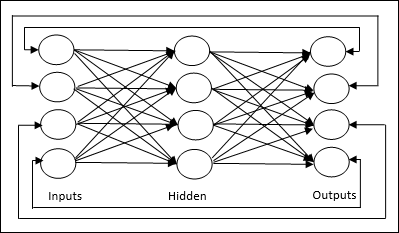## Adjustments of Weights or Learning

Learning, in artificial neural network, is the method of modifying the weights of connections between the neurons of a specified network. Learning in ANN can be classified into three categories namely supervised learning, unsupervised learning, and reinforcement learning.

### Supervised Learning

As the name suggests, this type of learning is done under the supervision of a teacher. This learning process is dependent.

During the training of ANN under supervised learning, the input vector is presented to the network, which will give an output vector. This output vector is compared with the desired output vector. An error signal is generated, if there is a difference between the actual output and the desired output vector. On the basis of this error signal, the weights are adjusted until the actual output is matched with the desired output.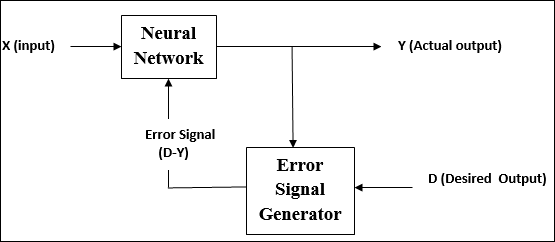### Unsupervised Learning

As the name suggests, this type of learning is done without the supervision of a teacher. This learning process is independent.

During the training of ANN under unsupervised learning, the input vectors of similar type are combined to form clusters. When a new input pattern is applied, then the neural network gives an output response indicating the class to which the input pattern belongs.

There is no feedback from the environment as to what should be the desired output and if it is correct or incorrect. Hence, in this type of learning, the network itself must discover the patterns and features from the input data, and the relation for the input data over the output.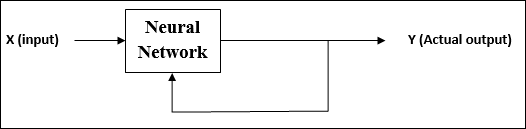### Reinforcement Learning

As the name suggests, this type of learning is used to reinforce or strengthen the network over some critic information. This learning process is similar to supervised learning, however we might have very less information.

During the training of network under reinforcement learning, the network receives some feedback from the environment. This makes it somewhat similar to supervised learning. However, the feedback obtained here is evaluative not instructive, which means there is no teacher as in supervised learning. After receiving the feedback, the network performs adjustments of the weights to get better critic information in future.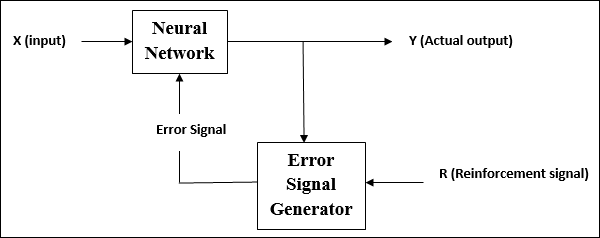## Activation Functions

It may be defined as the extra force or effort applied over the input to obtain an exact output. In ANN, we can also apply activation functions over the input to get the exact output. Followings are some activation functions of interest −

### Linear Activation Function

It is also called the identity function as it performs no input editing. It can be defined as −

$$F(x)\:=\:x$$

### Sigmoid Activation Function

It is of two type as follows −

• Binary sigmoidal function − This activation function performs input editing between 0 and 1. It is positive in nature. It is always bounded, which means its output cannot be less than 0 and more than 1. It is also strictly increasing in nature, which means more the input higher would be the output. It can be defined as

$$F(x)\:=\:sigm(x)\:=\:\frac{1}{1\:+\:exp(-x)}$$

• Bipolar sigmoidal function − This activation function performs input editing between -1 and 1. It can be positive or negative in nature. It is always bounded, which means its output cannot be less than -1 and more than 1. It is also strictly increasing in nature like sigmoid function. It can be defined as

$$F(x)\:=\:sigm(x)\:=\:\frac{2}{1\:+\:exp(-x)}\:-\:1\:=\:\frac{1\:-\:exp(x)}{1\:+\:exp(x)}$$